NCERT Solutions for Class 6 Maths Chapter 5 Understanding Elementary Shapes Ex 5.8 are part of NCERT Solutions for Class 6 Maths. Here we have given NCERT Solutions for Class 6 Maths Chapter 5 Understanding Elementary Shapes Ex 5.8.

 Board CBSE Textbook NCERT Class Class 6 Subject Maths Chapter Chapter 5 Chapter Name Understanding Elementary Shapes Exercise Ex 5.8 Number of Questions Solved 45 Category NCERT Solutions

## NCERT Solutions for Class 6 Maths Chapter 5 Understanding Elementary Shapes Ex 5.8

Question 1.
Examine whether the following are polygons. If anyone among them is not, say why?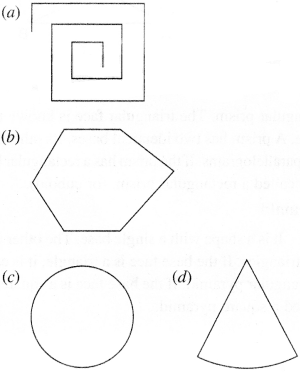Solution :
(a) is not a closed figure and hence is not a polygon.
(b) is a polygon of six sides.
(c) is not a polygon since it is not made of line segments.
(d) is not a polygon since it is not made of line segments.

Question 2.
Name each polygon: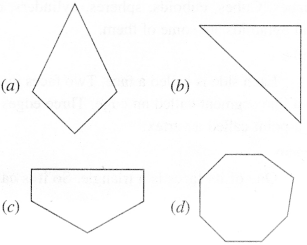Make two more examples of each of these.
Solution :
(b) A Triangle
(c) A Pentagon (5-sided)
(d) An Octagon (8-sided).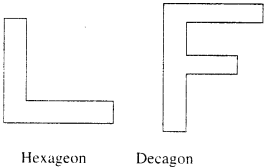Question 3.
Draw a rough sketch of a regular hexagon. Connecting any three of its vertices, draw a triangle. Identify the type of the triangle you have drawn.
Solution :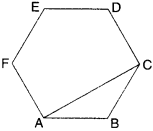The triangle ABC drawn is an obtuse-angled isosceles triangle.

Question 4.
Draw a rough sketch of a regular octagon. (Use squared paper if you wish). Draw a rectangle by joining exactly four of the vertices of the octagon.
Solution :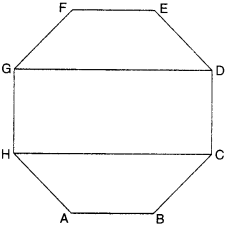Question 5.
A diagonal is a line segment that joins any two vertices of the polygon and is not a side of the polygon. Draw a rough sketch of a pentagon and draw its diagonals.
Solution :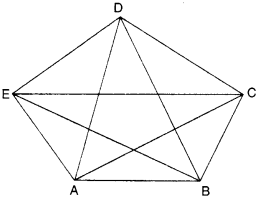We hope the NCERT Solutions for Class 6 Maths Chapter 5 Understanding Elementary Shapes Ex 5.8 help you. If you have any query regarding NCERT Solutions for Class 6 Maths Chapter 5 Understanding Elementary Shapes Ex 5.8, drop a comment below and we will get back to you at the earliest.